# Law of corresponding states

(Redirected from Corresponding states)

The law of corresponding states is an empirical law which encapsulates the finding that the equations of state for many real gases are remarkably similar when they are expressed in terms of reduced temperatures (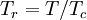$T_r = T/T_c$), pressures, (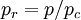$p_r = p/p_c$) and volumes (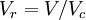$V_r = V/V_c$), where the subscript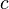$c$ represents the value of the property at the critical point. This law was first described by Johannes Diderik van der Waals in his 1873 thesis, and forms the subject of a paper by him in 1913 .

## Assumptions

Pitzer  produced a list of assumptions in order for the law of corresponding states to apply. This list was later modified by Guggenheim . These are:

1. There is negligible difference between Fermi–Dirac statistics and Bose–Einstein statistics for the system (i.e. the system behaves classically).
2. The effect of quantisation of the translational degrees of freedom is negligible (i.e. the system behaves classically).
3. The molecules are spherically symmetrical, either actually or by virtue of rapid and free rotation.
4. The intramolecular degrees of freedom are assumed to be completely independent of the volume per molecule.
5. The potential energy will be taken as a function only of the various intermolecular distances.
6. The potential energy for a pair of molecules can be written as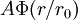$A\Phi (r/r_0)$ where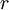$r$ is the intermolecular distance, and$A$ and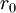$r_0$ are characteristic constants, and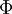$\Phi$ is a universal function.

## Examples

For argon, krypton, nitrogen, oxygen, carbon dioxide and methane one has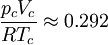$\frac{p_cV_c}{RT_c}\approx 0.292$

(for pressure measured in atmospheres, and volume in cm3mole-1)

For neon, argon, and oxygen one has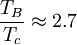$\frac{T_B}{T_c} \approx 2.7$

where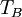$T_B$ is the Boyle temperature.

For neon, argon, krypton,and xenon one has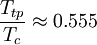$\frac{T_{tp}}{T_c} \approx 0.555$

where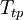$T_{tp}$ is the triple point.

## Acentric factor

The acentric factor  is defined in terms of the vapour pressure at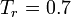$T_r = 0.7$. It has been shown that a number of substances have the behavior if they share the same acentric factor.

## Colloids

The law of corresponding states has been extended to suspensions of spherical colloids that interact via a pair potential by Noro and Frenkel .